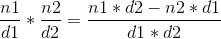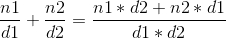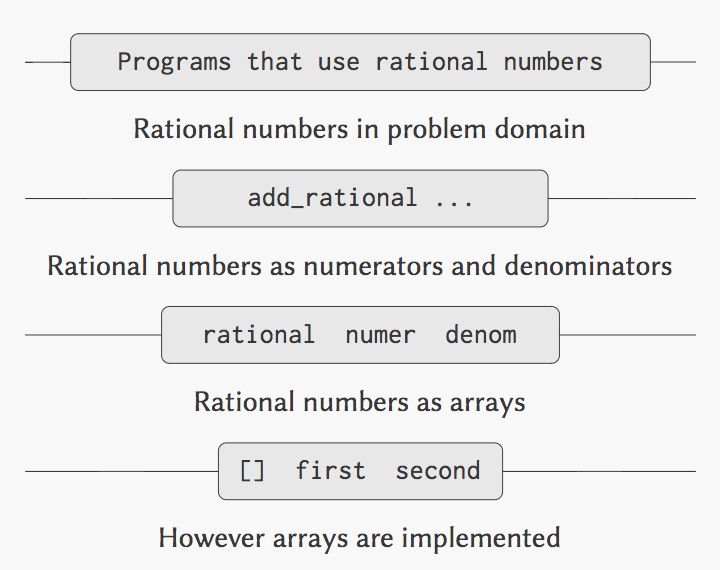# Ruby SICP - Abstraction Barriers

yfractal · 2017年06月11日 · 1812 次阅读

## 优美的代码（Beautiful Code）

SCIP 里面也讲了一种，就是 Build Abstraction Barriers。

Clean Code 也有类似的建议

Don't Mix Different Levels of Abstractions。

## 上需求## 上代码

### 实现实数操作

``````def add_rational(x, y)
numer_x, denom_x =  numer(x), denom(x)
numer_y, denom_y =  numer(y), denom(y)

rational(numer_x * denom_y + numer_y * denom_x, denom_x * denom_y)
end

def multiply_rational(x, y)
rational(numer(x) * numer(y), denom(x) * denom(y))

def rational_eq?(x, y)
numer(x) * denom(y) == numer(y) * denom(x)

``````

### 实现 constructor 和 selectors

``````def rational(numer, denom)
[numer, denom]
end

def numerator(x)
x.first
end

def denominator(x)
x.second
end

``````

### 这真的好吗？

So easy，

``````# Improved constructor
def rational(n, d)
gcd = n.gcd(d)
[n / gcd, d / gcd]
end
``````### 使用 Ruby 的类来实现

``````class Rational
# 构建 Rational
def initialize(numer, denom)
xxx
end

# getter 方法
def number
@numer
end

def multiply_rational
xxx
end
end
``````